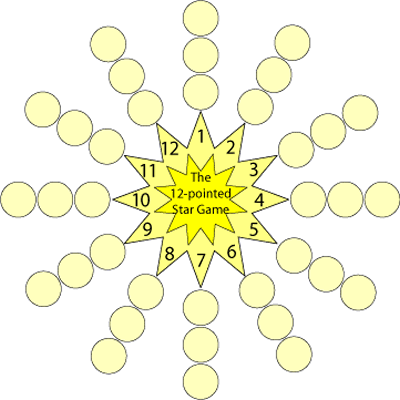#### You may also like### Prompt Cards

These two group activities use mathematical reasoning - one is numerical, one geometric.### Consecutive Numbers

An investigation involving adding and subtracting sets of consecutive numbers. Lots to find out, lots to explore.### Exploring Wild & Wonderful Number Patterns

EWWNP means Exploring Wild and Wonderful Number Patterns Created by Yourself! Investigate what happens if we create number patterns using some simple rules.

# The Twelve Pointed Star Game

##### Age 7 to 11 Challenge Level:

The game is for two or more players.
Each player chooses one, two or three numbers.
Players then take it in turns to roll two dice and add the scores.

The player who has chosen that number puts a counter on the appropriate circle.  (So for example I'm playing with my friend Zac. I choose $2$, $4$ and $6$, Zac chooses $7$, $8$ and $9$. Zac rolls the dice and it's a $4$ and a $2$ - so I can put a counter on $6$.)

The winner is the first player to have counters on all three circles belonging to one of their chosen numbers.Play the game a few times.
Here is a copy of the star which you can print off and use.

Which are good numbers to choose? Why?
Which are poor numbers to choose? Why?
Which is the worst number to choose? Why?